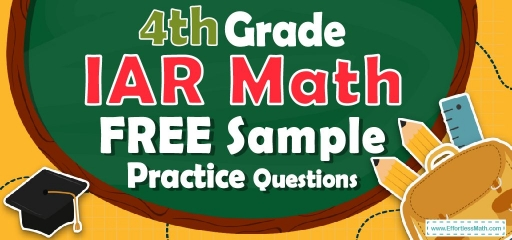# 4th Grade IAR Math FREE Sample Practice QuestionsIf your 4th-grade student wants to take the IAR math test, do not miss our Illinois Assessment of Readiness (IAR) sample math practice questions! This is exactly what you need to measure the readiness of a 4th-grade student.

Using sample practice questions before taking the IAR math test will help your student relax more on the day of the test. This sample practice question is a collection of the most important and common questions that 4th-grade students will face in the IAR math test.

Find out the strengths and weaknesses of your 4th-grade student using our IAR math sample practice questions!

It will be possible for your 4th-grade students to be fully prepared to take the IAR 2022 math test after using the sample practice questions.

Do not miss the related links at the bottom of this post! Because with the help of these links, you can gain a better understanding of the needs of your 4th-grade student for the IAR math test.

The Absolute Best Book to Ace 4th Grade IAR Math Test

## 10 Sample 4th Grade IAR Math Practice Questions

1- What is the value of A in the equation $$64 ÷ A = 8$$

A. $$2$$

B. $$4$$

C. $$6$$

D. $$8$$

2- Jason’s favorite sports team has won $$0.62$$ of its games this season. How can Jason express this decimal as a fraction?

A. $$\frac{6}{2}$$

B.$$\frac{62}{10}$$

C. $$\frac{62}{100}$$

D. $$\frac{6.2}{10}$$

3- Use the models below to answer the question.
$$\img{https://appmanager.effortlessmath.com/public/images/questions/taar422d.JPG}$$
Which statement about the models is true?

A. Each shows the same fraction because they are the same size.

B. Each shows a different fraction because they are different shapes.

C. Each shows the same fraction because they both have $$3$$ sections shaded.

D. Each shows a different fraction because they both have $$3$$ shaded sections but a different number of total sections.

4- Sophia flew $$2,448$$ miles from Los Angeles to New York City. What is the number of miles Sophia flew rounded to the nearest thousand?

A. $$2,000$$

B. $$2,400$$

C. $$2,500$$

D. $$3,000$$

5- Write $$\frac{124}{1000}$$ as a decimal number.

A. $$1.24$$

B. $$0.124$$

C. $$12.4$$

D. $$0.0124$$

6- Erik made $$12$$ cups of juice. He drinks $$3$$ cups of juice each day. How many days will Erik take to drink all of the juice he made?

A. $$2$$ days

B. $$4$$ days

C. $$8$$ days

D. $$9$$ days

7- Jason has prepared $$\frac{4}{10}$$ of his assignment. Which decimal represents the part of the assignment Jason has prepared?

A. $$4.10$$

B. $$4.01$$

C. $$0.4$$

D. $$0.04$$

8- Emma described a number using these clues.
• 3 digits of the number are $$4, 7$$, and $$9$$
• The value of the digit $$4$$ is $$(4 × 10)$$
• The value of the digit $$7$$ is $$(7 × 1000)$$
• The value of the digit $$9$$ is $$(9 × 10000)$$
Which number could fit Emma’s description?

A. $$9,724.04$$

B. $$90,734.40$$

C. $$97,040.04$$

D. $$98,740.70$$

9- There are $$18$$ boxes and each box contains $$26$$ pencils. How many pencils are in the boxed-in total?

A. $$108$$

B. $$208$$

C. $$468$$

D. Not here

10- Emily and Ava were working on a group project last week. They completed $$\frac{7}{10}$$ of their project on Tuesday and the rest on Wednesday.
Ava completed $$\frac{3}{10}$$ of their project on Tuesday. What fraction of the group project did Emily complete on Tuesday?

A. $$\frac{2}{10}$$

B. $$\frac{3}{10}$$

C. $$\frac{4}{10}$$

D. $$\frac{5}{10}$$

Best 4th Grade IAR Math Workbook Resource

1- D
$$A = 64 ÷ 8$$
$$A=8$$

2- C
$$0.62$$ is equal to $$\frac{62}{100}$$

3- D
The model for the first fraction is divided into $$6$$ equal parts. We shade 3_6 to show the same amount as 1_2. The model for the second fraction is divided into $$8$$ equal parts. We shade 3_ 8 that it shows these two models are different fractions.

4- A
When rounding to the nearest thousand, you will need to look at the last three digits. If the last three digits are $$449$$ or less round to the next number that is smaller than the number given and ends with three zeros.
On the other hand, If the last three digits are $$500$$ or more, round to the next number bigger than the given number and end with three zeros.

5- B
$$\frac{124}{1000}$$ is equal to $$0.124$$.

6- B
$$12 ÷ 3 = 4$$

7- C
$$\frac{4}{10}=0.4$$

8- C
Only choice C fits Emma’s description

9- C
$$18 × 26 = 468$$

10- C
$$\frac{7}{10}-\frac{3}{10}=\frac{4}{10}$$

The Best Books to Ace the 4th Grade IAR Math Test

### What people say about "4th Grade IAR Math FREE Sample Practice Questions - Effortless Math: We Help Students Learn to LOVE Mathematics"?

No one replied yet.

X
30% OFF

Limited time only!

Save Over 30%

SAVE $5 It was$16.99 now it is \$11.99